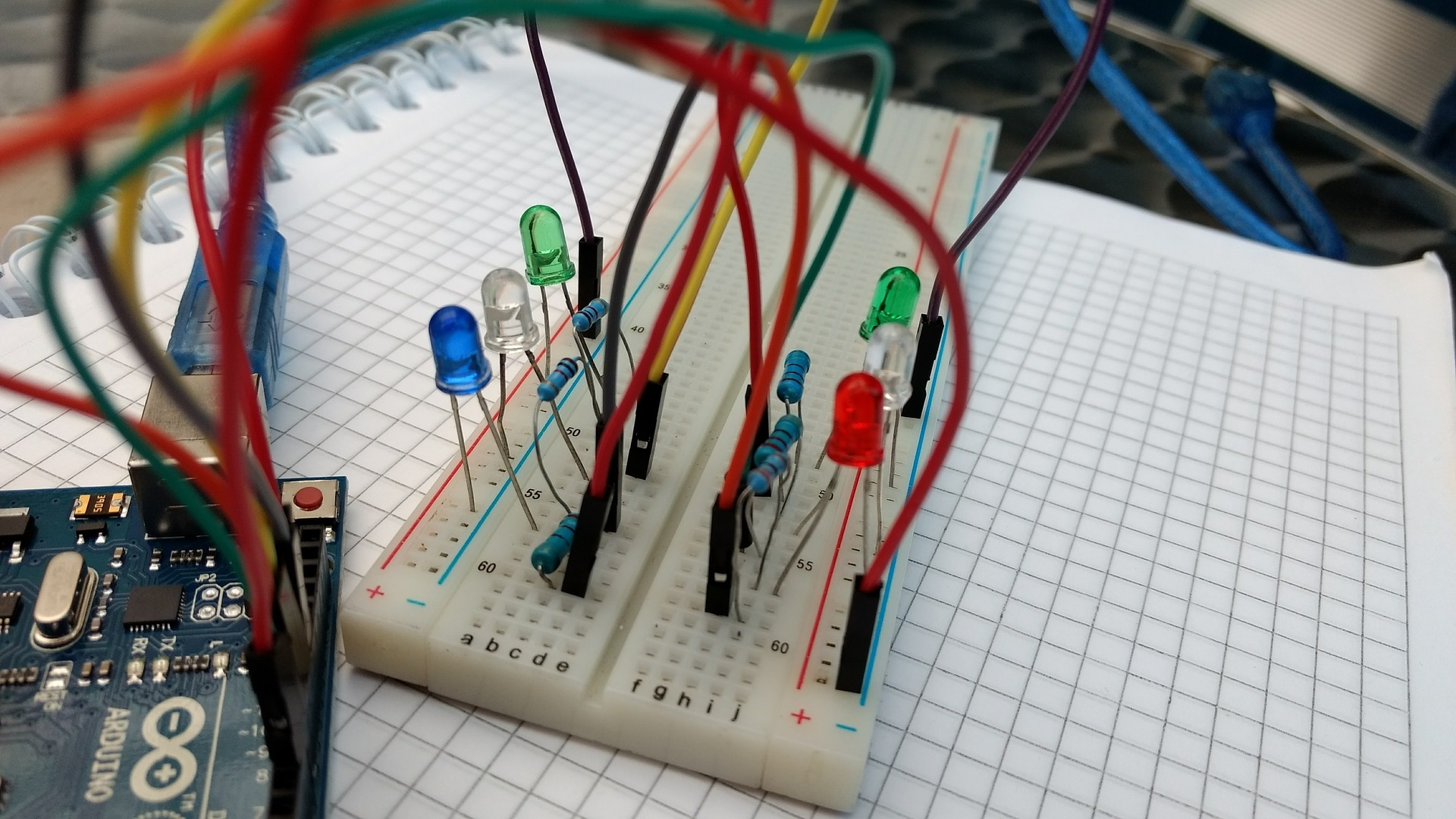## Romuxsim### How Electronics simulator Works?

This simulator is done in Javascript because it has to work in the browser. In simulation it parses the diagram and creates a system of equations describing the voltage and current at each point in the diagram. The voltage equations are basically "Ohm's Law" and the current equations are "Kirchoff's Law". Once we have the equations, We need to solve the equations to know the values. it is implemented by linear equation solver. Finally we have a unique values of each nodes and update these values to draw this circuit.